# GSEB Solutions Class 10 Maths Chapter 15 Probability Ex 15.1

Gujarat Board GSEB Solutions Class 10 Maths Chapter 15 Probability Ex 15.1 Textbook Questions and Answers.

## Gujarat Board Textbook Solutions Class 10 Maths Chapter 15 Probability Ex 15.1

Question 1.
Complete the following statements:

1. Probability of an event E + Probability of the event ‘not E’ = ………..
2. The probability of an event that cannot happen is ……… Such an event is called
3. Probability of an event that is certain to happen is …….. Such an event is called
4. The sum of the probabilities of all the elementary events of an experiment is
5. The probability of an event is greater than or equal to and less than or equal to

Solution:

1. 1
2. 0, impossible event
3. 1, a sure or certain event
4. 1
5. 0, 1

Question 2.
Which of the following experiments have likely outcomes? Explain.

1. A driver attempts to start a car. The car starts or does not start.
2. A player attempts to shoot a basketball. She/he shoots or misses the shoot.
4. A baby is born. It is a boy or a girl.

Solution:

1. Unequally outcome
2. Unequally outcome
3. Equally likely outcome
4. Equally likely outcome

Question 3.
Why is tossing a coin considered to be a fair way of deciding which team should get the ball at the beginning of a football game?
Solution:
In the experiment of tossing a coin, the possible outcomes (Head and Tail) are equally likely. Hence, both the teams get an equal chance to get the ball.Question 4.
which of the following cannot be the probability of an event?
(a) $$\frac {2}{3}$$
(b) -1.5
(c) 15%
(d) 0.7
Solution:
(b) -1.5 cannot be the probability of an event as 0 ≤ P(E) ≥ 1.

Question 5.
If P(E) = 0.05, what is the probability of‘not E’?
Solution:
P(E) + P(not E) = 1
⇒ 0.05 + P(not E) = 1
⇒ P(not E) = 1 – 0.05 = 0.95

Question 6.
A bag contains lemon-flavored candies only. Malini takes out one candy without looking into the bag. What is the probability that she takes out
1. an orange-flavored candy?
2. a lemon-flavored candy? (CBSE 2012)
Solution:
1. As the bag contains only lemon-flavored candies, the probability that she takes out an orange-flavored candy is ‘0’ [Impossible event]

2. As the bag contains only lemon-flavored candies, the probability that she takes out a lemon-flavored candy is ‘1’. [Sure or certain event]Question 7.
It is given that in a group of 3 students, the probability of 2 students not having the same birthday is 0.992. what is the probability that the 2 students have the same birthday ?
Solution:
P[2 students have the same birthday]
= 1 – P[2 students not having the same birthday]
= 1 – 0.992 = 0.008

Question 8.
A bag contains 3 red balls and 5 black balls. A balls is drawn at random from the bag. what is the probability that the ball drawn is (i) red (ii) not red?
Solution:
Number of red balls = 3
Number of black balls = 5
Total number of balls = 3 + 5 = 8
(i) P(a red ball) = $$\frac {3}{8}$$
(ii) P(Not red balls) = 1 – P(red ball) = 1 – $$\frac {3}{8}$$ = $$\frac {5}{8}$$

Question 9.
A box contains 5 red marbles, 8 white marbles and 4 green marbles. One marble is taken out of the box at random. What is the probability that the marble is taken out will be (i) red (ii) white (iii) not green?
Solution:
Number of red marbles = 5
Number of white marbles = 8
Number of green marbles = 4
Total number of marbles = 5 + 8 + 4 = 17
⇒ Number of all possible outcomes = 17
Number of outcomes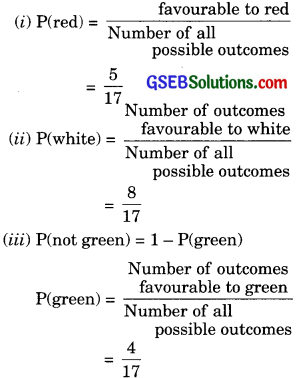∴ P(not green) = 1 – $$\frac {4}{17}$$ = $$\frac {13}{17}$$Question 10.
A piggy bank contains a hundred 50 p coins, fifty ₹ 1 coins, twenty ₹ 2 coins and ten ₹ 5 coins. If it is equally likely that one of the coins will fall odd when the bank is turned upside down, what is the probability that the coin (i) will be of a 50 p coin? (ii) will not be a ₹ 5 coin?
Solution:
Number of 50 p coins in the piggy bank = 100
Number ₹ 1 coins in the piggy bank = 50
Number of ₹ 2 coins = 20
Number of ₹ 5 coins = 10
∴ Total number of coins = 100 + 50 + 20 + 10 = 180
(i) P(50 p com) = $$\frac {100}{180}$$ = $$\frac {5}{9}$$
(ii) P(not ₹ 5 coin) = 1 – P(₹ 5 coin) = 1 – $$\frac {10}{180}$$ = $$\frac {17}{18}$$

Question 11.
Gopi buys a fish from a shop for his aquarium. The shopkeeper takes out one fish at random from a bank containing 5 male fish and 8 female fish (see figure). What is the probability that the fish taken out is a male fish?Solution:
Number of male fish = 5
Number of female fish = 8
Total number of fishes = 5 + 8 = 13
P(male fish) = $$\frac {5}{13}$$Question 12.
A game of chance consists of spinning an arrow that comes to rest pointing at one of the numbers 1, 2, 3, 4, 5, 6, 7, 8 (see figure), and these are equally likely outcomes. What is the probability that it will point at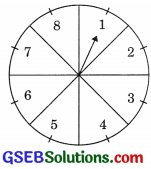1. 8?
2. and odd numbers?
3. a number greater than 2?
4. a number less than 9?
Solution:
Total number of outcomes = 8
1. P(8) = $$\frac {1}{8}$$
2.  Odd numbers are 1, 3, 5, 7 i.e. 4 in number.
∴ P(an odd number) = $$\frac {4}{8}$$ = $$\frac {1}{2}$$

3. Numbers greater than 2 are 3, 4, 5, 6, 7, 8 i.e. 6 in numbers
∴ P(number greater than 2) = $$\frac {6}{8}$$ = $$\frac {3}{4}$$

4. Since of all the numbers are less than 9
∴ P(number less than 9) = $$\frac {8}{8}$$ =1 [Certain event]Question 13.
A die is thrown once. Find the probability of getting
1. a prime number
2. a number lying between 2 and 6
3. an odd number
Solution:
When a die is thrown, the number of all possible outcome {1, 2, 3, 4, 5, 6} are 6.
1. Prime numbers are 2, 3, 5 i.e. 3 in number.
∴ P(a prime number) = $$\frac {3}{6}$$ = $$\frac {1}{2}$$

2. Number lying between 2 and 6 are 3, 4, 5 i.e. 3 in number.
∴ P(a number lying between 2 and 6) = $$\frac {3}{6}$$ = $$\frac {1}{2}$$

3. Odd numbers are 1, 3, 5 i.e. 3 in number.
∴ P(an odd number) = $$\frac {3}{6}$$ = $$\frac {1}{2}$$

Question 14.
One card is drawn from a well shuffled deck of 52 cards. Find the probability of getting:

1. a king of red colour
2. a face card
3. a red face card
4. the jack of hearts
6. the queen of diamonds. (CSBE 2012)

Solution:
Total number of cards in a deck = 52

1. P(a king of red colour) = $$\frac {2}{52}$$ = $$\frac {1}{26}$$
2. P(a face card) = $$\frac {12}{52}$$ = $$\frac {3}{13}$$
3. P(a red face card) = $$\frac {6}{52}$$ = $$\frac {3}{26}$$
4. P(the jack of hearts) = $$\frac {1}{52}$$
5. P(a spade) = $$\frac {13}{52}$$ = $$\frac {1}{4}$$
6. P(the queen of diamonds) = $$\frac {1}{52}$$Question 15.
Five cards-the ten, jack, queen, king and ace of diamonds, are well-shuffled with their face downwards. One card is then picked up at random.
(i) What is the probability that the card is the queen?
(ii) If the queen is drawn and put aside, what is the probability that the second card picked up is (a) an ace? (b) a queen?
Solution:
Total number of cards = 5
(i) P(queen) = $$\frac {1}{5}$$
(ii) Now total number of cards left = 4
(a) P(an ace) = $$\frac {1}{4}$$
(b) P(queen) = $$\frac {0}{4}$$ = 0

Question 16.
12 defective pens are accidentally mixed with 132 good ones. It is not possible just look at a pen and tell whether or not it is defective. One pen is taken out at random from this lot. Determine the probability that the pen is taken out is a good one. (CBSE 2012)
Solution:
Number of defective pens = 12
Number of good pens = 132
Total number of pens = 12 + 132 = 144
P(Good one) = $$\frac {132}{144}$$ = $$\frac {11}{12}$$Question 17.
(i) A lot of 20 bulbs contain 4 defective ones. One bulb is drawn at random from the lot. What is the probability that this bulb is defective?
(ii) Suppose the bulb is drawn in (i) is not defective and is not replaced. Now one bulb is drawn at random from the rest. What is the probability that this bulb not defective?
Solution:
Total number of bulbs = 20
Number of defective bulbs = 4
(i) P (defective bulb) = $$\frac {4}{20}$$ = $$\frac {1}{5}$$
(ii) Now, the total number of bulbs left = 19
Number of defective bulbs = 4
P(not defective) = $$\frac {15}{19}$$

Question 18.
A box contains 90 discs which are numbered from 1 to 90. If one disc is drawn at random from the box, find the probability that it bears:

1. a two-digit number.
2. a perfect square number.
3. a number divisible by 5.

Solution:
1. Total discs = 90
Favourable cases are 10, 11, 12, 13, … 90 = 81
P(a two-digit number) = $$\frac {81}{90}$$ = $$\frac {9}{10}$$

2. Favourable cases are 1, 4, 9, 16, 25, 36, 49, 64, 81, i.e. = 9
P(a perfect square number) = $$\frac {9}{90}$$ = $$\frac {1}{10}$$

3. Favourable cases are 5, 10, 15, 20, … 90 i.e. = 18
P(a number divisible by 5) = $$\frac {18}{90}$$ = $$\frac {1}{5}$$Question 19.
A child has a die whose six faces show the letters as given below: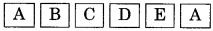The die is thrown once. What is the probability of getting
(i) A? (ii) D?
Solution:
Total faces = 6
(i)P(A) = $$\frac {2}{6}$$ = $$\frac {1}{3}$$
(ii) P(D) = $$\frac {1}{6}$$

Question 20.
Suppose you drop a die at random on the rectangular region shown in Figure. What is the probability that it will land inside the circle with diameter 1 m?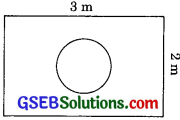Solution:
Area of circle = πr2
= π($$\frac {1}{2}$$)2 = $$\frac {π}{4}$$ m2
Area of rectangle = 3 x 2 = 6 m2
∴ P(die will land inside the circle)

Question 21.
A lot consists of 144 ball pens of which 20 are defective and the others are good. Nuri will buy a pen if it is good, but will not buy if it is defective. The shopkeeper draws one pen at random and gives it to her. What is the probability that:
(ii) she will not buy it?
Solution:
Number of defective ball pens = 20
Total number of pens = 144
Number of good ball pens = 144 – 20 = 124
(i) P(she will buy it) = P(good) = $$\frac {124}{144}$$ = $$\frac {31}{36}$$
(ii) P(she will not buy it) = P(defective) = $$\frac {20}{144}$$ = $$\frac {5}{36}$$Question 22.
1. Two dice, one blue and one grey are thrown at the same time. Complete the following table: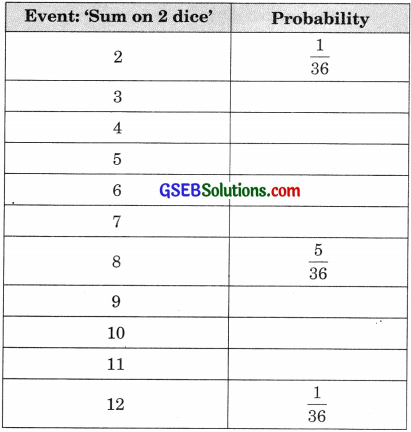2. A student argues that ‘there are 11 possible outcomes 2, 3, 4, 5, 6, 7, 8, 9, 10, 11, and 12. Therefore, each of them has a probability $$\frac {1}{11}$$ Do you agree with this argument? Justify your answer.
Solution:
1. Two dice can be thrown as 6 x 6 = 36 ways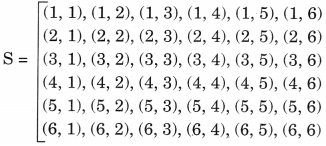P(a total of 2) = $$\frac {1}{36}$$,
Favorable cases are (1, 1)
P(a total of 3) = $$\frac {2}{36}$$
Favorable cases are (1, 2) (2, 1)
P(a total of 4) = $$\frac {3}{36}$$,
Favorable cases are (1, 3), (3, 1), (2, 2)
P(a total of 5) = $$\frac {4}{36}$$
Favourable cases are (1, 4), (4,1), (2, 3), (3, 2)
P(a total of 6) = $$\frac {5}{36}$$,
Favourable cases are (1, 5), (5, 1), (2, 4), (4, 2), (3, 3)
P(a total of 7) = $$\frac {6}{36}$$
Favourable cases are (1, 6), (6, 1), (2, 5), (5, 2), (3, 4), (4, 3)
P(a total of 8) = $$\frac {5}{36}$$
Favorable cases are (2, 6), (6, 2), (3, 5), (5, 3), (4, 4)
P(a total of 9) = $$\frac {4}{36}$$
Favorable cases are (3, 6), (6, 3), (4, 5), (5,4)
P(a total of 10) = $$\frac {3}{36}$$
Favorable cases are (4, 6), (6, 4), (5, 5)
P(a total of 11) = $$\frac {2}{36}$$
Favorable cases are (6, 5), (5, 6)
P(a total of 12) = $$\frac {1}{36}$$
Favorable cases are (6, 6)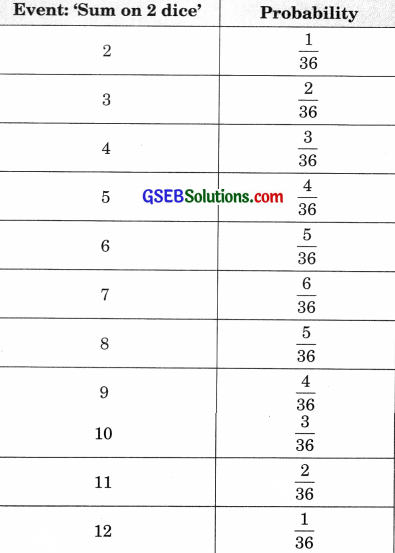2. We have P(sum 2) = $$\frac {1}{36}$$
P(Sum 3) = $$\frac {2}{36}$$ and so on.
∴ No, the eleven sums are not equally likely. Thus, the given statement is not correct.Question 23.
A game consists of tossing a one rupee coin 3 times and noting its outcome each time. Hanif wins if all the tosses give the same result i.e., three heads or three tails, and loses otherwise. Calculate the probability that Hanif will lose the game.
Solution:
S = [HHH, HHT, HTH, THH, TTH, THT, HTT, TTT]
∴ n(S) = 8
P(Hanif wins) = P(3H or 3T)
= $$\frac {1}{8}$$ + $$\frac {1}{8}$$ = $$\frac {2}{8}$$ = $$\frac {1}{4}$$
P(Hanif loses) = 1 – $$\frac {1}{4}$$ = $$\frac {3}{4}$$

Question 24.
A die is thrown twice. What is the probability that
1. 5 will not come up either time ?
2. 5 will come up at least once ?
[Hint. Throwing a die twice and throwing two dice simultaneously are treated as the same experiment.]
Solution:
A die is thrown twice.
∴ Total no. of ways = 6 x 6 = 36 ways
Favourable coins are (1, 5), (2, 5), (3, 5), (4, 5), (5, 5), (6, 5), (5,1), (5, 2), (5, 3), (5, 4), (5, 6) = 11 ways
Unfavourable ways are: 36 – 11 = 25
1. P(5 will not come up either time) = $$\frac {25}{36}$$
2. P(5 will not come up atleast once) = $$\frac {11}{36}$$Question 25.
Which of the following arguments are correct and which are not correct? Give reasons for your answer.
1. If two coins are tossed simultaneously there are three possible outcomes – two heads, two tails or one of each. Therefore, for each of these outcomes, the probability is $$\frac {1}{3}$$

2. If a die is thrown, there are two possible outcomes an odd number or an even number. Therefore, the probability of getting an odd number is $$\frac {1}{2}$$
Solution:
1. S – [HH, HT, TH, TT];  n(S) = 4
P(2 Heads) = $$\frac {1}{4}$$
P(2 Tails) = $$\frac {1}{4}$$
P(one each) = $$\frac {2}{4}$$
∴ Given statement is incorrect.

2. S = [1, 2, 3, 4, 5, 6]; n(S) = 6
P(odd numbers) = $$\frac {3}{6}$$ = $$\frac {1}{2}$$
P(even numbers) = $$\frac {3}{6}$$ = $$\frac {1}{2}$$
∴ Given statement is correct.